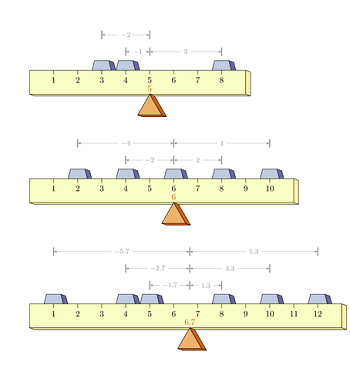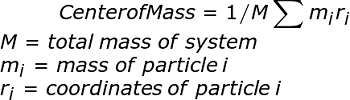# Calculating Center of Mass: Definition, Equation & Example

Lesson Transcript
Instructor
Sharon Linde

Sharon has an Masters of Science in Mathematics and a Masters in Education

Expert Contributor
Kathryn Boddie

Kathryn has taught high school or university mathematics for over 10 years. She has a Ph.D. in Applied Mathematics from the University of Wisconsin-Milwaukee, an M.S. in Mathematics from Florida State University, and a B.S. in Mathematics from the University of Wisconsin-Madison.

What is the center of mass and how is it calculated? Is it the same as the centroid or center of gravity? Learn how to find the center of mass of objects in one-, two-, even three-dimensional space! Updated: 04/26/2020

## Definition of Center of Mass

Before we get started, let's get some terms out of the way. To start, we need to know the center of mass for an object, or group of objects, is the point about which the entire mass of the system is equally distributed. Next to know is the centroid, which is the geometric center of a given shape. For instance, the exact center of a sphere is also the centroid of that shape. Finally, center of gravity refers to the point about which the forces of gravity are balanced.

Although the center of mass, the centroid, and the center of gravity often coincide, they are all different concepts. The centroid is equal to the center of mass only when mass distribution is uniform (always the same). For example, in a ball filled with air, the centroid and center of mass will be the same. Replacing the bottom half with a metal plug would significantly change the center of mass, but would not change the centroid. Meanwhile, the center of gravity and the center of mass are only equal when the entire system is subject to a uniform gravitational field.See in the image how the center of mass changes as the weights are moved around, but the centroid of the shape is always at the center of the ruler? That's how this works.

An error occurred trying to load this video.

Try refreshing the page, or contact customer support.

Coming up next: What Is a Vector? - Definition & Types

### You're on a roll. Keep up the good work!

Replay
Your next lesson will play in 10 seconds
• 0:05 Definition of Center of Mass
• 1:15 Equation for Center of Mass
• 2:08 Example 1 - Two…
• 3:14 Example 2 - Three…
• 4:37 Example 3 - Four…
• 6:57 Lesson Summary
Save Save

Want to watch this again later?

Log in or sign up to add this lesson to a Custom Course.

Timeline
Autoplay
Autoplay
Speed Speed

## Equation for Center of Mass

The equation for finding the center of mass for an object is:This equation can be used for vectors of any dimension and solved using appropriately sized matrices. However, for small numbers of particles and dimensions, it's usually easier to apply this equation to each coordinate separately, as we'll see in the examples following this section.

You shouldn't be scared of the summation in the above equation. For a two mass system along a single dimension, this complicated looking equation would reduce to this:

Center of Mass = {(m1*r1) + (m2*r2)} / (m1 + m2)

## Example 1 - Two Objects in a Line

Let's say you have a one-yard rod of negligible weight with one ball at each end. If one of the balls weighs 6 pounds and the other weighs 2 pounds, where along the rod would it be possible to balance the system? This situation reduces the three-dimensional concept of center of mass down to a single dimension. Crazy, right?

Wait, how can we proceed with a center of mass calculations if we have weights instead of masses? Since the force of gravity is the same for both objects here, the center of mass and the center of gravity is the same. There are some systems where this is not the case, such as satellites or planetary bodies with eccentric orbits, but it works perfectly fine in this instance.

Using our equation and taking x=0 as the center of the heavier ball, we get:

x = (6 lb)(0 ft)+(2 lb)(3 ft)/(6 lb+2 lb) = (0 + 6)ft lb / 8 lb = 0.75 ft

## Example 2 - Three Objects on a Plane

Let's try another. Determine the center of mass for 3 objects on a flat board with the following masses and locations on the coordinate plane, in meters:

A: 2 kg, (0, 10)

B: 3 kg, (10, 1)

C: 7 kg, (2, 2)

This example steps up the complexity one notch. We now have to consider two dimensions, (x, y).

x-coordinate center of mass =

2 kg(0 m) + 3 kg(10 m) + 7 kg(2 m) / (2 kg+3 kg+7 kg) = (0+30+14)kg m / 12 kg

= 3.67 m

y-coordinate center of mass =

2 kg(10 m) + 3 kg(1 m) + 7 kg(2 m) / (2 kg+3 kg+7 kg) = (20+3+14)kg m / 12 kg

= 3.08 m

The center of mass for this system is (3.67 m, 3.08 m). Starting to make more sense? Let's try one more.

To unlock this lesson you must be a Study.com Member.
Create your account

Additional Activities

## Problems on Centre of Mass

In the following examples, students will apply their knowledge of how to calculate the center of mass of objects in spaces of various dimensions. Some examples will be straightforward calculations in different dimensional spaces and one example will require students to solve for an unknown location of one of the objects in the space given the center of mass. After completing the examples, students will be more comfortable with the formula for calculating the center of mass and how to use it.

### Questions

Round answers to the nearest hundredth if needed.

1) Find the center of mass of two objects placed on the ends of a straight rod of length 9 inches if the objects weigh 3 pounds and 7 pounds.

2) Find the center of mass of three objects in the xy-plane with the following masses and locations on the plane in centimeters: 9 kg at (3,2), 4 kg at (1,7), and 2 kg at (-2, 0).

3) If the center of mass of three objects in the xy-plane is given by the location (3 cm, 4 cm) and two of the objects have the following mass and locations: 5 kg at (3,2), 2 kg at (1, 3) and the third object has mass 8 kg, find the location of the third object.

### Solutions

1) We will say the location of the 3 pound object is at 0 and the location of the 7 pound object is at 9. Then, the center of mass is given by ((3 lb * 0 in + 7 lb * 9 in)) / (3 lb + 7lb) = 63 in lb /10 lb = 6.3 inches.

2) The x-coordinate of the center of mass is given by (9 kg * 3 cm + 4 kg * 1 cm + 2 kg * -2 cm) / (9 + 4 + 2 kg) = 27 kg cm / 15 kg = 1.8 cm.

The y-coordinate is calculated similarly as (9 kg * 2 cm + 4 kg * 7 cm + 2 kg * 0 cm) / (9 + 4 + 2 kg) = 46 kg cm / 15 kg = 46 / 15 cm or about 3.07 cm.

The center of mass is at approximately (1.8 cm, 3.07 cm).

3) Start with the x-coordinate of the center of mass. We have 3 cm = (5 kg * 3 cm + 2 kg * 1 cm + 8 kg * x cm) / (5 + 2 + 8 kg) and so simplifying, we have 3 cm = (17 + 8x) / 15 cm. Solving for x, we have 45 cm = 17 + 8x cm, 28 cm = 8x cm, and so x = 3.5 cm.

Similarly for the y-coordinate, we have 4 cm = (5 kg * 2 cm + 2 kg * 3 cm + 8 kg * y cm) / (5 + 2 + 8 kg) and so simplifying, we have 4 cm = (16 + 8y) / 15 cm. Solving for y, we have 60 cm = 16 + 8y cm, 44 cm = 8y cm, and so y = 5.5 cm.

Thus the third object is located at (3.5, 5.5).

### Register to view this lesson

Are you a student or a teacher?

### Unlock Your Education

#### See for yourself why 30 million people use Study.com

##### Become a Study.com member and start learning now.
Back
What teachers are saying about Study.com
Create an account to start this course today
Used by over 30 million students worldwide